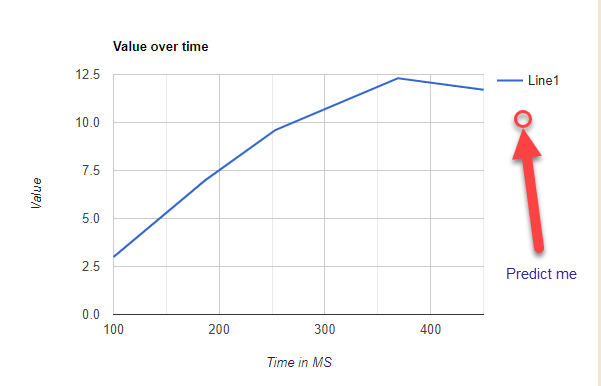# Vector3 Exptrapolation algorithm

I’m looking for a Vector3 extrapolation algorithm. I’m working on a networked multiplayer game and am trying to do lag compensation on networked VR player movement. I’ve tried doing a simple projection based on last position and current position to get next position, but the results aren’t great. What I need is, an algorithm that takes in several of the last position coordinates, and uses that to make an educated guess on a position at n milliseconds in the future. A method signature like this

Vector3 predictionPosition = PredictNextPosition(List previousPositions, int MSToNextPosition)

To break it down to it’s most simple form, given a value over time, predict the value at set time in the future. Here’s a high-tech graph for you all to enjoy. Any help would be appreciated, thank you.If you have the start and end point of the vector, you can work out any point along the line using the formula: `startVector + endVector * distance` where distance could be the time.

For example, if I used the position last frame, and the position this frame, I could work out the position a couple milliseconds later like this:

``````float PredictionTime;
Vector3 lastPos = new Vector3();

void Update()
{
Vector3 pos = transform.position;
Vector3 futurePos = lastPos + pos * PredictionTime;

lastPos = transform.position;
}
``````

I’m not sure it will work perfectly, as it might need some tweaking. Hope it helps. @Slobdell

You can use Polynomial Interpolation. I know you need extrapolation but that works the same way. Note that you also need to give the time for each control point, otherwise the time parameter would make no sense. You can’t extrapolate X milliseconds into the future if you don’t know when the previous points happened. Here is an implementation using the Lagrange base:

``````struct ControlPoint {
public Vector3 position;
public float time;
}

Vector3 Extrapolate(List<ControlPoint> points, float time) {
Vector3 result = Vector3.zero;

for (int j = 0; j < points.Count; j++) {
float weight = 1;
ControlPoint pj = points[j];

for (int k = 0; k < points.Count; k++) {
if (j != k) {
ControlPoint pk = points[k];
weight *= (time - pk.time) / (pj.time - pk.time);
}
}

result += weight * pj.position;
}

return result;
}
``````

One thing to keep in mind is that more control points isn’t necessarily better with this technique as it might give too much importance to not so relevant data. Polynomial interpolation finds a function that interpolates precisely between all control points so if there is one bad outlier then that can ruin the extrapolation. You might need to find a way of picking a handful of well distributed points (e.g. three points distributed evenly over the last 0.5 seconds or something like that).